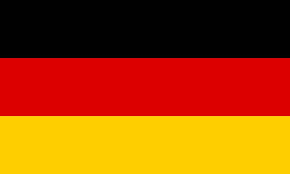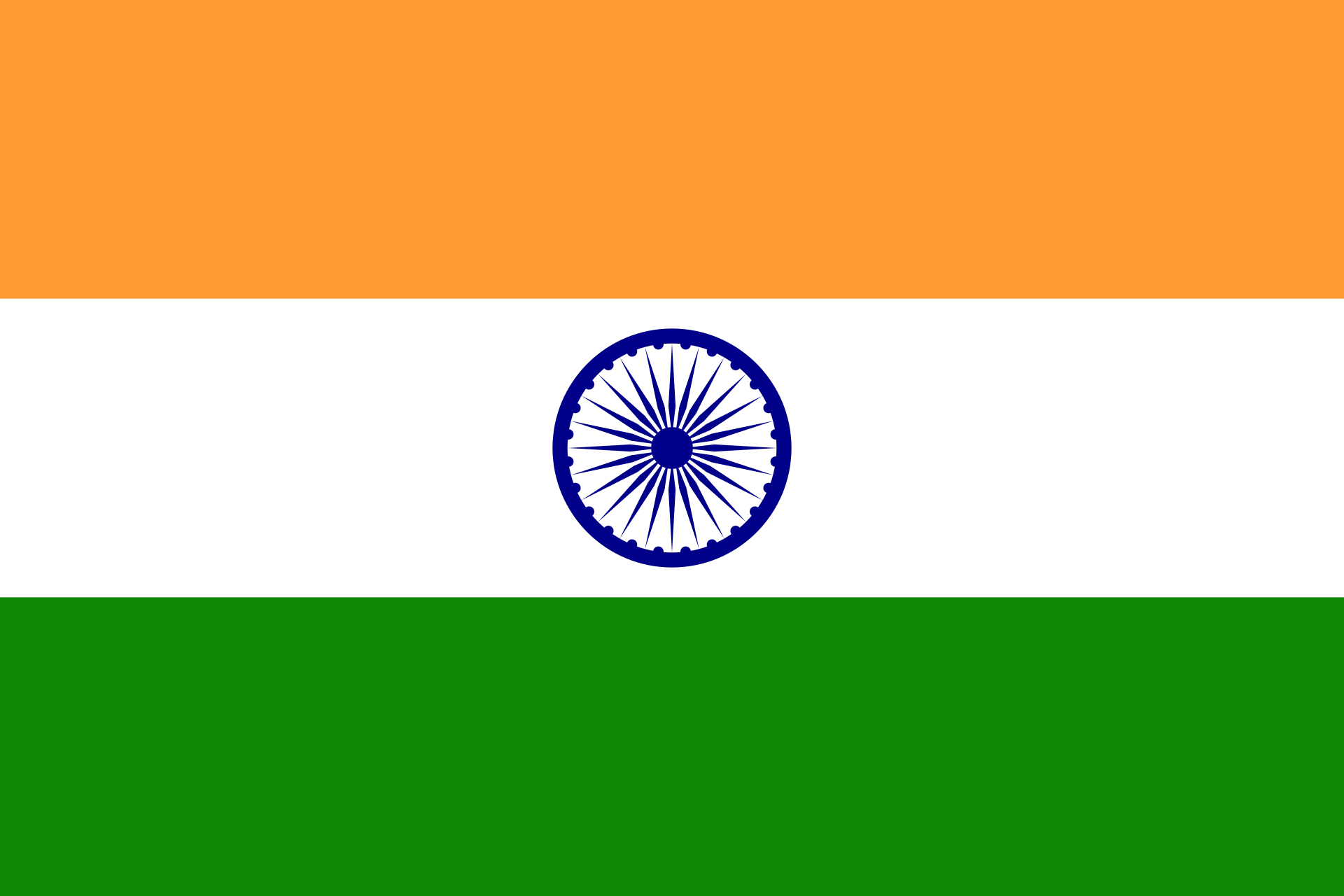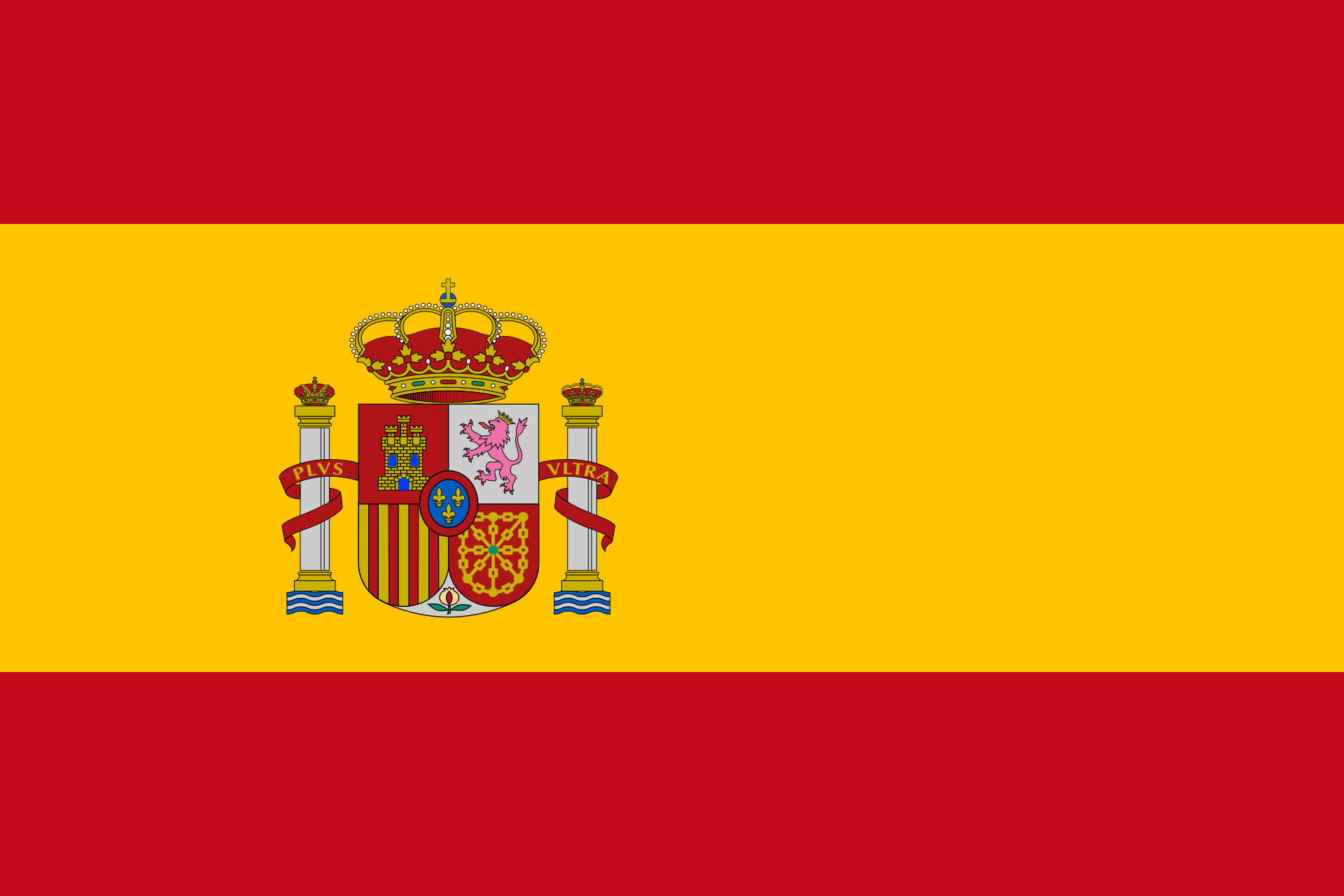# Fraction calculatorThis calculator adds / subtracts fractions.
 + -
Fraction calculator for mixed numbers

 + -
Multiplying / dividing fractions

 • ∶
Multiplying / dividing mixed numbers

 • ∶

This calculator transforms fractions into decimal numbers and vice versa

Enter a fraction (e.g. 2/3) or a decimal number. Mathepower converts it.

Hints:

If you enter 3/7 , you getIf you enter 1 4/5 , you get
 1 4 5

If you enter 0,_3 , you get   0,3  = 0, 333333....
If you enter 3,42_56 , you get   3.4256

The sign _ says that all further signs are below the overline.

## What are fractions needed for?

First an example: Imaginepersons want to eat a cake. How much cake does everyone get? Of course, the calculation is. But what is the result? The answer is simply to create a new number called(speak it: one fourth). If you want to imagine how much this is, just draw a cake and part it into four pieces.

## And what is such a fraction?

What we just did with the cake, can be done with every number: Imagine you want to divide two numbers, but it is not possible. Then, you just make up a new number that shall be the result of this division. So the fractionmeans nothing else than the result of. The number above is called numerator, the lower one denominator. Sohas numeratorand denominator.

## And how to calculate with such fractions?

First keep in mind: There are different looking fractions describing the same number. For example,andare both the same as, causeandboth give. So we have two fractions looking different but describing the same number. Transforming a fraction into another that describes the same number is called expanding or cancelling the fraction.

If you want to add or subtract fractions, you have to put them on the same denominator. This can be done by finding the least common multiple of the denominators.

If you want to multiply fractions, just multiply the numerators and the denominators. To divide fractions, interchange the numerator and the denominator of the fraction you want to divide by, then multiply those fractions

## How does one call the numbers containing fractions?

Those are the rational numbers.